Next: Axisymmetric Stokes Flow Up: Incompressible Viscous Flow Previous: Lubrication Theory

# Stokes Flow

Steady flow in which the viscous force density in the fluid greatly exceeds the advective inertia per unit volume is generally known as Stokes flow, in honor of George Stokes (1819-1903). Because, by definition, the Reynolds number of a fluid is the typical ratio of the advective inertia per unit volume to the viscous force density (see Section 1.16), Stokes flow implies Reynolds numbers that are much less than unity. In the time independent, low Reynolds number limit, Equations (10.1) and (10.2) reduce to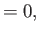(10.80)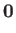(10.81)

It follows from these equations that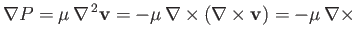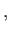(10.82)

where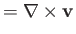, and use has been made of Equation (A.177). Taking the curl of this expression, we obtain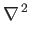(10.83)

which is the governing equation for Stokes flow. Here, use has been made of Equations (A.173), (A.176), and (A.177).Next: Axisymmetric Stokes Flow Up: Incompressible Viscous Flow Previous: Lubrication Theory
Richard Fitzpatrick 2016-03-31